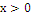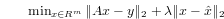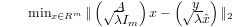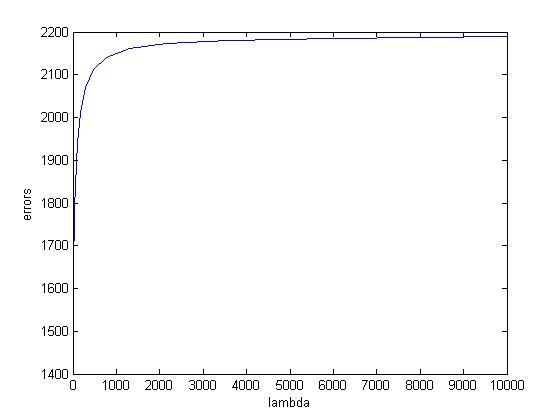## a

We impose a constraintto have meaningful results, since every entry in x represents a travel time on a link of the network.

## b

In general, the matrix A may not be full column rank, therefore the closed-form solution cannot be applied. We remedy the normal least-square equation by applying the so-called regularized least squares. We solve the following problem instead:For reasonable values of lambda, this formulation generates an approximation to the actual travel times which is near the observed mean travel times, but is adjusted by the measurements from the current time-step. This problem can be reformulated as a least-squares problem as follows:Observe that the two norms are independent, given x.

## c

Numerical Implementation